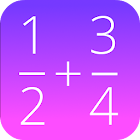Fraction Calculator

• SEARCH TYPE

All Android applications categories

All Android games categoriesFraction Calculator

by: 1.7K 6.4

6.4 Users
rating

Screenshots

Description

This free math calculator lets you add, subtract, multiply and divide fractions. You can use common fractions (like 1/2) and mixed fractions (like 2 4/5).

Best mathematical tool for school and college! If you are a student, it will helps you to learn arithmetics and calculations with fraction numbers.

Note: A common fraction consists of an integer numerator, displayed above a line (or before a slash in this app), and a non-zero integer denominator, displayed below (or after the slash) that line. Numerators and denominators are also used in fractions that are not common, including compound fractions, complex fractions, and mixed numerals.

Tags: fraction calculator , adding fractions calculator , adding subtracting multiplying and dividing fractions , calculator for subtracting fractions , frações solver , how to add subtract multiply and divide fractions , démo gratuit sur les fractions , 分数 計算 乗算 , code divide fraction calculator

Users review

from 1.659 reviews

"Good"

6.4